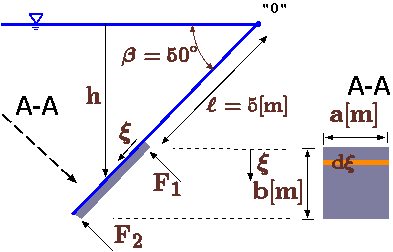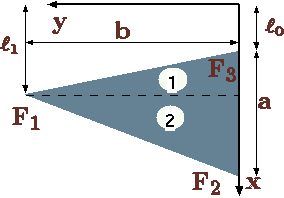# 4.5.1: Fluid Forces on Straight Surfaces

A motivation is needed before going through the routine of derivations. Initially, a simple case will be examined. Later, how the calculations can be simplified will be shown.

Example 4.14

Consider a rectangular shape gate as shown in Figure 4.20. Calculate the minimum forces, $$F_1$$ and $$F_2$$ to maintain the gate in position. Assuming that the atmospheric pressure can be ignored.

Solution 4.14Fig. 4.20 Rectangular area under pressure.

The forces can be calculated by looking at the moment around point "O.'' The element of moment is $$a\,d\xi$$ for the width of the gate and is
$dM = \overbrace{P\, \underbrace{a\, d\xi}_{dA}}^{dF} (ll + \xi)$
The pressure, $$P$$ can be expressed as a function $$\xi$$ as the following
\begin{align*}
P = g\, \rho \, (ll +\xi) sin \beta
\end{align*}
The liquid total moment on the gate is
\begin{align*}
M = \int_0^b g\, \rho \, (ll +\xi) \sin \beta\, a\, d\xi (ll + \xi)
\end{align*}
The integral can be simplified as
\begin{align*}
M = g\, a\,\rho\,\sin\beta\,\int_0^b (ll +\xi)^2 d\xi
\end{align*}
The solution of the above integral is
\begin{align*}
M = g\, \rho\,a\,\sin\beta\,\left( \dfrac{3\,b\,{l}^{2}+3\,{b}^{2}\,l+{b}^{3}}{3} \right)
\end{align*}
This value provides the moment that $$F_1$$ and $$F_2$$ should extract. Additional equation is needed. It is the total force, which is
\begin{align*}
F_{total} = \int_0^b g\, \rho \, (ll +\xi) \sin \beta\, a\, d\xi
\end{align*}
The total force integration provides
\begin{align*}
F_{total} = g\,\rho\,a\,\sin \beta\, \int_0^b (ll +\xi) d\xi
= g\,\rho\,a\,\sin \beta\,\left(\dfrac{2\,b\,ll+{b}^{2}}{2}\right)
\end{align*}
The forces on the gate have to provide
\begin{align*}
F_1 + F_2 = g\,\rho\,a\,\sin \beta\,\left(\dfrac{2\,b\,ll+{b}^{2}}{2}\right)
\end{align*}
Additionally, the moment of forces around point "O'' is
\begin{align*}
F_1\,ll + F_2 (ll + b) =
g\, \rho\,a\,\sin\beta\,\left(
\dfrac{3\,b\,{l}^{2}+3\,{b}^{2}\,l+{b}^{3}}{3}
\right)
\end{align*}
The solution of these equations is
\begin{align*}
F_1 = \dfrac{\left( 3\,ll+b\right)
\,a\,b\,g\,\rho\,\sin\beta}{6}
\end{align*}
\begin{align*}
F_2 = \dfrac{\left( 3\,ll+2\,b\right)
\,a\,b\,g\,\rho\,\sin\beta}{6}
\end{align*}

Fig. 4.21. Schematic of submerged area to explain the center forces and moments.

The above calculations are time consuming and engineers always try to make life simpler. Looking at the above calculations, it can be observed that there is a moment of area in equation __?__ and also a center of area. These concepts have been introduced in Chapter 3. Several represented areas for which moment of inertia and center of area have been tabulated in Chapter 3. These tabulated values can be used to solve this kind of problems.

### Symmetrical Shapes

Consider the two–dimensional symmetrical area that are under pressure as shown in Figure 4.21. They symmetry is around any axes parallel to axis $$x$$. The total force and moment that the liquid extracting on the area need to be calculated. First, the force is $F = \int_{A} PdA = \int \left(P_{atmos} + \rho g h \right) dA = AP_{atmos} + \rho g \int_{ll_{0}}^{ll_{1}} \left(\xi + ll_{0}\right)sin\beta dA \tag{139}$ In this case, the atmospheric pressure can include any additional liquid layer above layer touching'' area. The atmospheric'' pressure can be set to zero. The boundaries of the integral of equation (139) refer to starting point and ending points not to the start area and end area. The integral in equation 139 can be further developed as $F_{total} = AP_{atmos} + \rho g sin\beta \left(ll_{0}A + \int_{ll_{0}}^{ll_{1}} \xi dA\right) \tag{140}$ In a final form as

Total Force in Inclined Surface

$F_{total} = A\left[P_{atmos} + \rho g sin\beta \left(ll_{0} + x_{c}\right)\right] \tag{141}$

Fig. 4.22. The general forces acting on submerged area.

The moment of the liquid on the area around point O'' is $M_{y} = \int_{\xi_{0}}^{\xi_{1}} P\left(\xi\right)\xi dA \tag{142}$ $M_{y} = \int_{\xi_{0}}^{\xi_{1}} \left(P_{atmos} + g\rho h \left(\xi\right)\right)\xi dA \tag{143}$ Or separating the parts as $M_{y} = P_{atmos} \int_{\xi_{0}}^{\xi_{1}}\xi dA + g\rho sin\beta \int_{xi_{0}}^{xi_{1}} \xi^{2}dA \tag{144}$ The moment of inertia, $$I_{x'x'}$$, is about the axis through point O'' into the page. Equation 144 can be written in more compact form as

Total Moment in Inclined Surface

$M_{y} = P_{atmos}x_{c}A + g\rho sin \beta I_{x'x'} \tag{145}$

Example 4.14 can be generalised to solve any two forces needed to balance the area/gate. Consider the general symmetrical body shown in figure 4.22 which has two forces that balance the body. Equations 141 and 145 can be combined the moment and force acting on the general area. If the atmospheric pressure'' can be zero or include additional layer of liquid. The forces balance reads $F_{1} + F_{2} = A\left[P_{atmos} + \rho g sin\beta \left(ll_{0} + x_{c}\right)\right] \tag{146}$ and moment balance reads $F_{1}a + F_{2}b = P_{atmos}x_{c}A + g\rho sin\beta I_{x'x'} \tag{147}$ The solution of these equations is $F_{1} = \frac{\left[\left(\rho sin\beta - \frac{P_{atmos}}{gb}\right)x_{c}+ll_{0}\rho sin\beta + \frac{P_{atmos}}{g}\right]bA}{g\left(b-a\right)} - \frac{I_{x'x'}\rho sin \beta}{g\left(b-a\right)} \tag{148}$ and $F_{2} = \frac{I_{x'x'}\rho sin \beta}{g\left(b-a\right)} - \frac{\left[\left(\rho sin\beta - \frac{P_{atmos}}{ga}\right)x_{c}+ll_{0}\rho sin\beta + \frac{P_{atmos}}{g}\right]aA}{g\left(b-a\right)} \tag{149}$ In the solution, the forces can be negative or positive, and the distance $$a$$ or $$b$$ can be positive or negative. Additionally, the atmospheric pressure can contain either an additional liquid layer above the touching'' area or even atmospheric pressure simply can be set up to zero. In symmetrical area only two forces are required since the moment is one dimensional. However, in non–symmetrical area there are two different moments and therefor three forces are required. Thus, additional equation is required. This equation is for the additional moment around the $$x$$ axis (see for explanation in Figure 4.23). The moment around the $$y$$ axis is given by equation 145 and the total force is given by 141. The moment around the $$x$$ axis (which was arbitrarily chosen) should be $M_{x} = \int_{A} y PdA \tag{150}$ Substituting the components for the pressure transforms equation 150 into $M_{x} = \int_{A} y \left(P_{atmos} + \rho g \xi sin \beta \right) dA \tag{151}$ The integral in equation 150 can be written as $M_{x} = P_{atmos} \int_{A} y dA + \rho g \xi sin \beta \int_{A} \xi y dA \tag{152}$ The compact form can be written as

Moment in Inclined Surface

$M_{x} = P_{atmos}Ay_{c} + \rho g sin\beta I_{x'y'} \tag{153}$

Fig. 4.23. The general forces acting on non symmetrical straight area.

The product of inertia was presented in Chapter 3. These equations (141), (145), and (153) provide the base for solving any problem for straight area under pressure with uniform density. There are many combinations of problems (e.g. two forces and moment) but no general solution is provided. Example to illustrate the use of these equations is provided.

Example 4.15

Calculate the forces which required to balance the triangular shape shown in the Figure 4.24.

Solution 4.15

The three equations that needs to be solved are
$F_1+F_2+F_3 = F_{total} \label{static:eq:triangleF1} \tag{154}$
The moment around $$x$$ axis is
$F_1\,b = M_y \label{static:eq:triangleMy} \tag{155}$
The moment around $$y$$ axis is
$F_1\,ll_1 +F_2\,(a +ll_0 ) +F_3\,ll_0 = M_x \label{static:eq:triangleMx} \tag{156}$
The right hand side of these equations are given before in equations (141), (145) and (153). The moment of inertia of the triangle around $$x$$ is made of two triangles (as shown in the Figure 4.24 for triangle 1 and 2). Triangle 1 can be calculated as the moment of inertia around its center which is $$ll_0+ 2*(ll_1-ll_0)/3$$. The height of triangle 1 is $$(ll_1−ll_0)$$ and its width $$b$$ and thus, moment of inertia about its center is $$I_{xx}=b(ll_1−ll_0)^{3}/36$$. The moment of inertia for triangle 1 about $$y$$ is
${I_{xx}}_1 = \dfrac{b (ll_1 - ll_0)^3}{36} + \overbrace{\dfrac{b (ll_1 - ll_0)}{3}}^{A_1} \, \overbrace{\left(ll_0+ \dfrac{2(ll_1-ll_0)}{3}\right)^2} ^{{\Delta x_1}^2} \tag{157}$ The height of the triangle 2 is $$a - (ll_1 - ll_0)$$ and its width $$b$$ and thus, the moment of inertia about its center is

${I_{xx}}_2 = \dfrac{b [a - (ll_1 - ll_0)]^3}{36} + \overbrace{\dfrac{b [a - (ll_1 - ll_0)]}{3}}^{A_2} \, \overbrace{\left(ll_1 + \dfrac{[a - (ll_1 - ll_0)]}{3} \right)^2}^{{\Delta x_2}^2} \tag{158}$Fig. 4.24 The general forces acting on a non symmetrical straight area.

and the total moment of inertia
$I_{xx} = {I_{xx}}_1 + {I_{xx}}_2$
The product of inertia of the triangle can be obtain by integration. It can be noticed that upper line of the triangle is $$y = \dfrac{\left(ll_1 - ll_0\right)x}{b} +ll_0$$. The lower line of the triangle is $$y = \dfrac{\left(ll_1 - ll_0 - a \right)x}{b} +ll_0 + a$$.
\begin{align*}
I_{xy} = \int_0^b \left[\int_{\dfrac{\left(ll_1 -
ll_0\right)x}{b} +ll_0}^{\dfrac{\left(ll_1 - ll_0 - a
\right)x}{b} +ll_0 + a} x\,y\, dx \right] dy
=\dfrac {2\,a\,b^2\,ll_1+2\,a\,b^2\,ll_0+a^2\,b^2} {24}
\end{align*}
The solution of this set equations is
\begin{align*}
F_1=\overbrace{\left[\dfrac{a\,b}{3}\right]}^{A}
\dfrac{\left( g\,\left( 6\,ll_1+3\,a\right)
+6\,g\,ll_0\right)
\,\rho\,\sin\beta+8\,P_{atmos}}{24},
\end{align*}
\begin{multline*}
\dfrac{F_2}
{\left[\dfrac{a\,b}{3}\right]}
=-
\begin{array}{c}
\dfrac{\left(
\left( 3\,ll_1-14\,a\right) - ll_0\,\left(
\dfrac{12\,ll_1}{a}-27\right) +
\dfrac{12\,{ll_0}^{2}}{a}\right)\,g
\,\rho\,\sin\beta}
{72}
- \\
\dfrac{\left( \left( \dfrac{24\,ll_1}{a}-24\right)
+\dfrac{48\,ll_0}{a}\right) \,P_{atmos}}
{72},
\end{array}
\end{multline*}
\begin{multline*}
\dfrac{F_3}
{\left[\dfrac{a\,b}{3}\right]} =
\begin{array}{c}
\dfrac{\left( \left(
a-\dfrac{15\,ll_1}{a}\right) +ll_0\,\left( 27-
\dfrac{12\,ll_1}{a}\right)
+\dfrac{12\,{ll_0}^{2}}{a}\right)\,g\,\rho\,\sin\beta }
{72}\\
+
\dfrac{\left( \left(\dfrac{24\,ll_1}{a}+24\right)
+\dfrac{48\,ll_0}{a}\right) \,P_{atmos} }{72}
\end{array}
\end{multline*}

## Contributors

• Dr. Genick Bar-Meir. Permission is granted to copy, distribute and/or modify this document under the terms of the GNU Free Documentation License, Version 1.2 or later or Potto license.